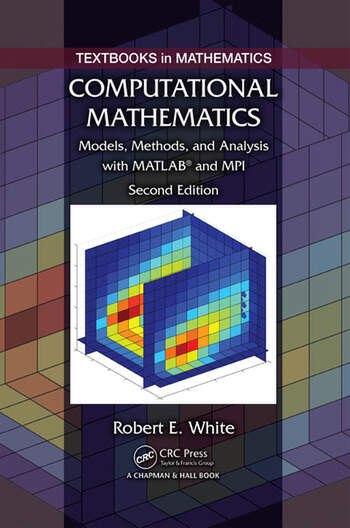# Computational Mathematics: Models, Methods, and Analysis with MATLAB® and MPI, Second Edition

## 2nd Edition

Robert E. White

Chapman and Hall/CRC
Published November 17, 2015
Textbook - 478 Pages - 97 B/W Illustrations
ISBN 9781482235159 - CAT# K23032
Series: Textbooks in Mathematics

For Instructors Request Inspection Copy

was \$120.00

USD\$96.00

SAVE ~\$24.00

FREE Standard Shipping!

## Preview

### Summary

Computational Mathematics: Models, Methods, and Analysis with MATLAB® and MPI is a unique book covering the concepts and techniques at the core of computational science. The author delivers a hands-on introduction to nonlinear, 2D, and 3D models; nonrectangular domains; systems of partial differential equations; and large algebraic problems requiring high-performance computing. The book shows how to apply a model, select a numerical method, implement computer simulations, and assess the ensuing results.

Providing a wealth of MATLAB, Fortran, and C++ code online for download, the Second Edition of this very popular text:

• Includes a new chapter with two sections on the finite element method, two sections on shallow water waves, and two sections on the driven cavity problem
• Introduces multiprocessor/multicore computers, parallel MATLAB, and message passing interface (MPI) in the chapter on high-performance computing
• Updates and adds code and documentation

Computational Mathematics: Models, Methods, and Analysis with MATLAB® and MPI, Second Edition is an ideal textbook for an undergraduate course taught to mathematics, computer science, and engineering students. By using code in practical ways, students take their first steps toward more sophisticated numerical modeling.

#### Instructors

We provide complimentary e-inspection copies of primary textbooks to instructors considering our books for course adoption.